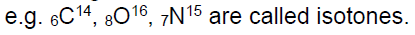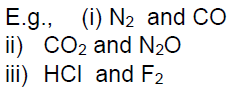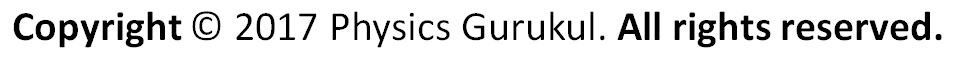# Atomic Models

We know the fundamental particles of the atom. Now let us see, how these particles are arranged in an atom to suggest a model of the atom.

## Thomson’s Model:

J.J. Thomson, in 1904, proposed that there was an equal and opposite positive charge enveloping the electrons in a matrix. This model is called the plum – pudding model after a type of Victorian dissert in which bits of plums were surrounded by matrix of pudding.This model could not satisfactorily explain the results of scattering experiment carried out by Rutherford who worked with Thomson.

## Rutherford’s Model:

α– particles emitted by radioactive substance were shown to be dipositive Helium ions
(He++) having a mass of 4 units and 2 units of positive charge.
Rutherford allowed a narrow beam of α–particles to fall on a very thin gold foil of thickness of the order of 0.0004 cm and determined the subsequent path of these particles with the help of a zinc sulphide fluorescent screen. The zinc sulphide screen gives off a visible flash of light when struck by an α- particle, as ZnS has the remarkable property of converting kinetic energy of α particle into visible light.

[For this experiment, Rutherford specifically used α particles because they are relatively heavy resulting in high momentum].

### Observation

i) Majority of the α–particles pass straight through the gold strip with little or no deflection.
ii) Some α–particles are deflected from their path and diverge.
iii) Very few α–particles are deflected backwards through angles greater than 90.
iv) Some were even scattered in the opposite direction at an angle of 180

[ Rutherford was very much surprised by it and remarked that “It was as incredible as if you fired a 15– inch shell at a piece of tissue paper and it came back and hit you”].

There is far less difference between air and bullet than there is between gold atoms and α-particles assuming of course that density of a gold atom is evenly distributed.

### Conclusions

1. The fact that most of the α – particles passed straight through the metal foil indicates the most part of the atom is empty.

2. The fact that few α – particles are deflected at large angles indicates the presence of a heavy positively charge body i.e., for such large deflections to occur α – particles must have come closer to or collided with a massive positively charged body.

3. The fact that one in 20,000 have deflected at 180° backwards indicates that volume occupied by this heavy positively charged body is very small in comparison to total volume of the atom.

### Atomic model

On the basis of the above observation, and having realized that the rebounding α-particles had met something even more massive than themselves inside the gold atom, Rutherford proposed an atomic model as follows.

i) All the protons (+ve charge) and the neutrons (neutral charge) i.e nearly the total mass of an atom is present in a very small region at the centre of the atom. The atom’s central core is called nucleus.

ii) The size of the nucleus is very small in comparison to the size of the atom. Diameter of the nucleus is about 10^–13cm while the atom has a diameter of the order of 10^–8 cm. So, the size of atom is 10^5 times more than that of nucleus.

iii) Most of the space outside the nucleus is empty.

iv) The electrons, equal in number to the net nuclear positive charge, revolve around the nucleus with fast speed in various circular orbits.

v) The centrifugal force arising due to the fast speed of an electron balances the coulombic force of attraction of the nucleus and the electron remains stable in its path.

Thus according to him atom consists of two parts (a) nucleus and (b) extra nuclear part.

### Defects of Rutherford’s atomic model:

1. Position of electrons: The exact positions of the electrons from the nucleus are not
mentioned
2. Stability of the atom: Neils Bohr pointed out that Rutherford’s atom should be highly unstable. According to the law of electrodynamics, the electron should therefore, continuously emit radiation and lose energy. As a result of this a moving electron will come closer and closer to the nucleus and after passing through a spiral path, it should ultimately fall into the nucleus.It was calculated that the electron should fall into the nucleus in less than 10^–8 sec. But it is known that electrons keep moving outside the nucleus.
To solve this problem Neils Bohr proposed an improved form of Rutherford’s atomic model.
Before going into the details of Neils Bohr model we would like to introduce you some important atomic terms.

### Atomic terms:

a) Atomic Number (Z): The atomic number of an element is the number of protons contained in the nucleus of the atom of that element.
b) Nucleons: Protons and neutrons are present in a nucleus. So, these fundamental particles are collectively known as nucleons.
c) Mass Number (A): The total number of protons and neutrons i.e, the number of nucleons present in the nucleus is called the mass number of the element.
d) Nuclide: Various species of atoms in general. A nuclide has specific value of atomic number and mass number.

### IUPAC notation of an atom (nuclide):

Let X be the symbol of the element. Its atomic number be Z and mass number be A.
Then the element can be represented as,e) Isotopes: Atoms of the element with same atomic number but different mass number.f) Isobars: Atoms having the same mass number but different atomic numbers,

e.g. P (15, 32) & S (16, 32) are called isobars.

g) Isotones: Atoms having the same number of neutrons but different number of protons or mass number.h) Isoelectronic: Atoms, molecules or ions having same number of electrons are isoelectronic.i) Nuclear isomer: Nuclear isomers (isomeric nuclei) are the atoms with the same atomic number and same mass number but with different radioactive properties.
Example of nuclear isomers is:
Uranium –X (half life 1.4 min) and
Uranium –Z (half life 6.7 hours)
The reason for nuclear isomerism is the different energy states of the two isomeric
nuclei. Other examples arej) Isosters: Molecules having same number of atoms and also same number of electrons
are called isosters.k) Atomic mass unit: Exactly equal to 1/12 of the mass of 6C12 atom
1 amu = 1.66 x 10^–27 kg = 931.5 MeVCBSE Class 11 Chemistry